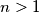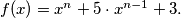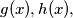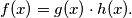### IMO Shortlist 1993 problem A7

Kvaliteta:
Avg: 0,0
Težina:
Avg: 9,0
Let$n > 1$ be an integer and let$f(x) = x^n + 5 \cdot x^{n-1} + 3.$ Prove that there do not exist polynomials$g(x),h(x),$ each having integer coefficients and degree at least one, such that$f(x) = g(x) \cdot h(x).$
Izvor: Međunarodna matematička olimpijada, shortlist 1993